# Periodic Classification of Elements Class 10 Important Questions Science Chapter 5

Solved the very best collection of Periodic Classification of Elements Class 10 Science Important Questions and Answers Chapter 5 Pdf from the latest NCERT edition books, It will help you in scoring more marks in CBSE Exams.

## Periodic Classification of Elements Class 10 Important Questions with Answers Science Chapter 5

### Periodic Classification of Elements Class 10 Important Questions Short Answer Type

Question 1.
What physical and chemical properties of elements were used by Mendeleev in creating his Periodic Table? List two observations which posed a challenge to Mendeleev’s Periodic Law.   (CBSE 2008)
The physical property used was the atomic mass of an element.
The chemical property used was the nature of oxide and hydride formed i.e., similarity in chemical properties were used by Mendeleev.

The two observations that posed challenge in Mendeleev Periodic Law were:

1. Arranging elements according to the increasing order of atomic mass could not be maintained. Chemical properties do not depend on atomic mass.
2. Isotopes were not given any place in the table as they have different atomic mass but same chemical properties.

Question 2.
Table given below shows a part of the Periodic Table.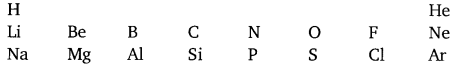Using this table explain why?
(a) Li and Na are considered as active metals.
(b) Atomic size of Mg is less than that of Na.
(c) Fluorine is more reactive than chlorine.   (Foreign 2008)
(a) Li and Na can readily lose electrons due to bigger size of atom.
(b) Mg has more number of protons than Na which attracts the electrons thereby reducing the size of Mg.
(c) Fluorine readily accepts/gains electrons to become F ion due to its small atomic size as compared to chlorine.

Question 3.
The position of three elements A, B and C in the Periodic Table are shown below: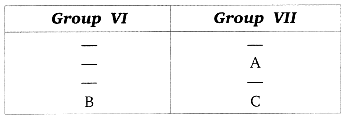Giving reasons, explain the following:
(а) Element A is a non-metal.
(б) Element B has a larger atomic size than element C
(c) Element C has a valency of 1.   (Foreign 2008)
(a) A is a non-metal because it can gain electrons easily, it has 7 valence electrons and form negative ions to become stable.
(b) The atomic number of B is less than C, it has less nuclear charge, less force of attraction between protons in the nucleus and valence electrons, hence its size is bigger than C.
(c) Element ‘C’ has 7 valence electrons, it can gain 1 electron to become stable so its valency is 1.

Question 4.
The position of three elements A, B and C in the Periodic Table are shown below:

 Period Group I II III 1 – – – 2 – – B 3 A C –

Giving reason, explain the following:
(a) Element A is a metal.
(b) Element C has a larger size than B.
(c) Element B has a valency of 3.   (Foreign 2008)
(a) ‘A’ is a metal because its valence electron is 1, it can readily lose electron to became stable.
(b) Element ‘C’ belongs to 3rd period it has 3 shells whereas ‘B’ has only 2 shells, it belongs to 2nd period, distance between nucleus and valence electrons is more in C, hence its size is bigger than B.
(c) ‘B’ belongs to III group, has 3 valence electrons, it can lose 3 electrons to become stable hence its valency is 3.

Question 5.
The elements of the second period of the Periodic Table are given below:

 Li Be B C N O F

(a) Give reason to explain why atomic radii decreases from Li to F.
(b) Identify the most
(i) Metallic and
(ii) Non-metallic element.  (AI, Foreign 2008)
(a) In a period on moving from left to right, the atomic number increases, the number of shells remains the same, nuclear charge increases the force of attraction on electrons towards the nucleus. The valence electrons are pulled at the centre, hence atomic radii decreases from Li to F.

(b) (i) Most metallic element → Li
(ii) Most non-metallic element → F.

Question 6.
(a) What is meant by periodicity in properties of elements with reference to the Periodic Table?
(b) Why do all the elements of the same group have similar properties?
(c) How will the tendency to gain electrons change as we go from left to right across a period? Why?   (AI 2009)
(a) The repetition of same properties after definite interval is called periodicity in properties.
(b) It is because all elements in a group have same valence electrons.
(c) Tendency to gain electrons increases from left to right in the period because the atomic size goes on decreasing and nuclear charge increases, which can attract the nearby electron.

Question 7.
An element ‘X’ has atomic number 13.
(a) Write its electronic configuration,
(b) State the group to which ‘X’ belongs.
(c) Is ‘X’ a metal or a non-metal?
(d) Write the formula of its bromide.   (CBSE 2012)
(a) 2, 8, 3
(b) Group 13
(c) ‘X’ is a metal.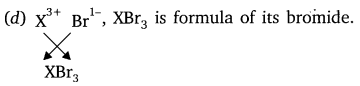Question 8.
State the Modern Periodic Law for classification of elements. How many
(a) Groups and
(b) periods are there in the Modem Periodic Table?    (CBSE 2012)
‘Properties of elements are the periodic function of their atomic number’.
(a) There are 18 groups and
(b) 7 periods in the Modern Periodic Table.

Question 9.
An element ‘M’ has atomic number 11.
(a) Write its electronic configuration,
(b) State the group to which ‘M’ belongs.
(c) Is ‘M’ a metal or a non-metal?
(d) Write the formula of its chloride.   (CBSE 2012)
(a) 2, 8, 1
(b) Group 1
(c) ‘M’ is a metal.Question 10.
An element ‘M’ has atomic number 12.
(a) Write its electronic configuration.
(b) State the group to which ‘M’ belongs,
(c) Is ‘M’ a metal or a non-metal?
(d) Write the formula of its oxide.   (CBSE 2012)
(a) 2, 8, 2
(b) Group 2
(c) M is a metal.Question 11.
The formula of magnesium oxide is MgO. State the formula of barium nitrate and barium sulphate, if barium belongs to the same group as magnesium.   (CBSE 2012)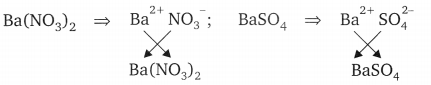Question 12.
Choose the answer from the following: Ca -20, Li -3, Na -11, Ne -10
(a) An element having two shells completely filled with electrons
(b) Two elements belonging to the same group of the periodic table.   (CBSE 2012)
(a) Ne -10 has electronic configuration as 2, 8 and its both the shells are completely filled.
(b) Elements Li -3 and Na -11 belong to the same group as both these elements has same valence electrons i.e., one and belong to group 1.

Question 13.
Why do all the elements of the
(a) same group have similar properties,
(b) same period have different properties? (CBSE 2012)
(a) Elements of same group have similar properties due to same number of valence electrons, that is, they have same valency.
(b) Elements of same period have different properties as they differ in number of valence electrons.

Question 14.
An element ‘E’ has following electronic configuration:

 K L M 2 8 6

(a) To which group of the periodic table does element ‘E’ belong?
(b) To which period of the periodic table does element ‘E’ belong?
(c) State the number of valence electrons present in element ‘E’.
(d) State the valency of the element ‘E’.   (CBSE 2012)
(a) ‘E’ belongs to group 16.
(b) It belongs to 3rd period.
(c) It has 6 valence electrons,
(d) Its valency is equal to 2.

Question 15.
An element has atomic number 13.
(a) What is the group and period number to which this element belongs?
(b) Is this element a metal or a non-metal? Justify your answer.   (CBSE 2012)
(a) It belongs to group 13 and 3rd period.
(b) It is a metal because it can lose 3 electrons to become stable.

Question 16.
The electronic configuration of two elements ‘A’ and ‘B’ are 2, 8, 7 and 2, 8, 8, 2, respectively. Write the atomic number of these elements. What will be formula of the compound formed and the nature of bond between them when these two elements chemically combine together?   (CBSE 2012)
‘A’ has atomic number 17. Its electronic configuration is 2, 8, 7. Its valency is equal to 1. ‘B’ has atomic number 20. Its electronic configuration is 2, 8, 8, 2. Its valency is 2.Ionic bond will be formed to form compound AB2.

Question 17.
The atomic numbers of three elements are given below:

 Element (symbol) A B C Atomic number 3 6 9

Write the symbol of the element which belongs to (a) group 1, (b) group 14, of the periodic table. State the period of the periodic table to which these elements belong. State reason to support your answer.   (CBSE 2012)
(a) A belongs to group 1.
(b) B belongs to group 14.
These elements belong to second period because these elements have two shells. A has electronic configuration 2, 1 and has one valence electron so, belongs to group 1. ‘B’ has electronic configuration 2, 4 and has four valence electrons so, belongs to group 14 and period 2. ‘C’ has electronic configuration 2, 7 and has seven valence electrons. All of them contain two shells and so belong to second period.

Question 18.
Na, Mg and Al are the elements having one, two and three valence electrons respectively. Which of these elements (a) has the largest atomic radius,
(b) is least reactive?
(a) Na has the largest atomic radius because on moving from Na to Al effective nuclear charge increases which causes decrease in atomic radius.
(b) Al is least reactive because it has smallest atomic size. It has greater effective nuclear charge, therefore, cannot lose electrons easily, hence it is least reactive.

Question 19.
(a) How are the following related?
(i) Number of valence electrons of different elements in the same group.
(ii) Number of shells of elements in the same period.
(b) How do the following change?
(i) Number of shells of elements as we go down a group.
(ii) Number of valence electrons of elements on moving from, left to right in a period.
(iii) Atomic radius in moving from left to right along a period.
(iv) Atomic size down a group. (CBSE 2012)
(a) (i) Different elements in same group have same number of valence electrons.
(ii) Number of shells of elements in same period are equal.

(b) (i) Number of shells of elements goes on increasing down the group.
(ii) Number of valence electrons of elements goes on increasing on moving from left to right in a period.
(iii) Atomic radius goes on decreasing on moving from left to right along a period.
(iv) Atomic size goes on increasing down a group.

Question 20.
(a) How many periods are there in the Modern Periodic Table of elements?
(b) How do atomic radius, valency and metallic character vary down a group?
(c) How do the atomic size and metallic character of elements vary as we move from left to right in a period?   (CBSE 2012)
(a) There are 7 periods.
(b) Atomic radius goes on increasing down the group. Valency remains same. Metallic character increases down the group.
(c) Atomic size decreases along a period from left to right. Metallic character decreases along a period from left to right.

Question 21.
How many groups and periods are there in the modem periodic table? How do the atomic size and metallic character of elements vary as we move:   (Delhi 2015)
(a) down a group, and
(b) from left to right in a period
18 groups, 7 periods
(a) Atomic size increases and metallic character increases.
(b) Atomic size decreases and metallic character decreases.

Question 22.
From the following elements :
4Be; 9F; 19K; 20Ca
(i) select the element having one electron in the outermost shell.
(ii) two elements of the same group.
Write the formula of and mention the nature of the compound formed by the union of 19K and element X(2, 8, 7).        (Delhi 2015, CBSE 2012)
(i) K/Potassium
(ii) Be and Ca.
KX or KCl is formed by union of K and X which is ionic/electrovalent in nature.

Question 23.
The atomic number of an element ‘X’ is 20.
(i) Determine the position of the element ‘X’ in the periodic table.
(ii) Write the formula of the compound formed when ‘X’ reacts/combines with another element ‘Y (atomic number 8).
(iii) What would be the nature (acidic or basic) of the compound formed ? Justify your answer.   (Foreign 2015)
Electronic configuration : 2, 8, 8, 2.
(i) ‘X’ is present in the 2nd group and 4th period of the periodic table.
(ii) Y (2, 6) has valency 2. Thus, an atom of Y accepts 2 electrons from X to form XY.
(iii) Basic because X is a metal and the oxides of metals are basic in nature. (Y, Atomic number = 8, oxygen)

Question 24.
Taking the example of an element of atomic number 16, explain how the electronic configuration of the atom of an element relates to its position in the modem periodic table and how valency of an element is calculated on the basis of its atomic number.   (AI 2015)
Electronic configuration of element with atomic no. 16 is 2, 8, 6.
Since it has 3 shells, the period no. will be 3.
Since the no. of valence electrons is 6, the group no. will be 10 + 6 = 16.
Valency of the element will be 8- valence electrons i.e., 8 – 6 = 2.

Question 25.
Two elements ‘P’ and ‘Q’ belong to the same period of the modern periodic table and are in Group-1 and Group-2 respectively. Compare their following characteristics in tabular form :   (AI 2015)
(a) The number of electrons in their atoms
(b) The sizes of their atoms
(c) Their metallic characters
(d) Their tendencies to lose electrons
(e) The formula of their oxides
(f) The formula of their chlorides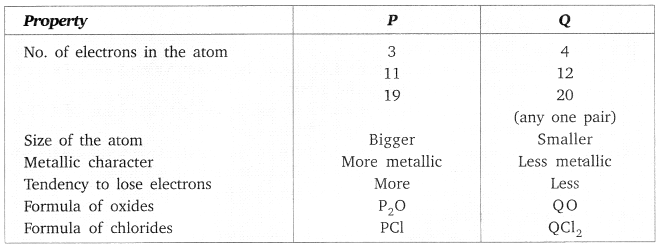Question 26.
Calcium is an element with atomic number 20. Stating reason answer each of the following questions:   (Delhi 2016)
(a) Is calcium a metal or non-metal?
(b) Will its atomic radius be larger or smaller than that of potassium with atomic number 19?
(c) Write the formula of its oxide.
(a) It is a metal. Since it has two electrons in its outermost shell/two valence electrons, which it can lose easily.
(b) K(19) is placed before Ca(20) in the same period/fourth period. Since the atomic radius decreases on moving from left to right along a period, the atomic radius of calcium is smaller than that of potassium.
(c) The formula of oxide of calcium is CaO, because the valency of calcium as well as that of oxygen is 2.

Question 27.
An element ‘M’ with electronic configuration (2, 8, 2) combines separately with (NO3), (SO4)2- and (PO4)3- radicals. Write the formula of the three compounds so formed. To which group and period of the Modern Periodic Table does the elements ‘M’ belong? Will ‘M’ form covalent or ionic compounds? Give reason to justify your answer. (Delhi 2016)

• The electronic configuration (2, 8, 2) of the element ‘M’ suggests that it belongs to group 2 and period 3 of the Modern Periodic Table and its valency is 2.
• The chemical formula of the compounds are
M(NO3)2/Mg(NO3)2; MSO4/MgSO4; M3(PO4)2 / Mg3(PO4)2.
• ‘M’ will form ionic compounds by losing two electrons.

Question 28.
Write the main aim of classifying elements. Name the basic property of elements used in the development of Modern Periodic Table. State the Modern Periodic Law. On which side (part) of the Modern Periodic Table do you find metals, metalloids and non-metals?   (Foreign 2016, 2015)
For systematic and simplified study of elements and their compounds.
Basic property: Atomic Number.

Modern periodic Law: The properties of elements are a periodic function of their atomic number.
Metals are found on the left side and centre of the Modern Periodic Table. Metalloids are found in a zig-zag manner between the metals and the non-metals. Non-metals are found on the right side of the Modern Periodic Table.

Question 29.
An element ‘X’ belongs to 3rd period and group 16 of the Modern Periodic Table.   (AI 2016)
(a) Determine the number of valence electrons and the valency of ‘X’.
(b) Molecular formula of the compound when ‘X’ reacts with hydrogen and write its electron dot structure.
(c) Name the element ‘X’ and state whether it is metallic or non-metallic.
(a) Electronic configuration of X – 2, 8, 6.
Valence electrons – 6
Valency = 8 – 6 = 2
(b) Formula with hydrogen – H2X or H2S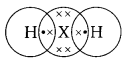(c) Sulphur; Non-Metal.

Question 30.
An element ‘X’ has mass number 35 and number of neutrons 18. Write atomic number and electronic configuration of ‘X’. Also write group number, period number and valency of ‘X’.    (AI 2016)
Atomic number of X = Mass number of X – No of neutrons = 35 – 18 = 17
Therefore Electronic configuration of X = 2, 8, 7
Group number =17
Period no = 3
Valency = 8 – 7 = 1

Question 31.
Choose from the following elements to answer the questions given below:
6C, 8O, 10Ne, 11Na, 14Si
(a) Elements that should be in the same period
(b) Elements that should be in the same group
State reason for the selection in each case.    (Delhi 2016)
The electronic configuration of all given elements is:
C = 2, 4
O = 2, 6
Ne = 2, 8
Na = 2, 8, 1
Si = 2, 8, 4
(a) Elements C, O and Ne are in the same period, as all of them have electrons occupying the first two shells. The elements Na and Si will be in the same 3rd period as both of them has electrons occupied in 3 shells.
(b) Elements C and Si will be in the same group because both these elements have same number of valence electrons that is 4.

### Periodic Classification of Elements Class 10 Important Questions Long Answer Type

Question 1.
On the basis of the table of Mendeleev’s Periodic Table
(a) Name the element which is in
(i) I group and 3rd period.
(ii) VII group and 2nd period.
(b) Suggest the formula for the following:
(i) Oxide of nitrogen
(ii) Hydride of oxygen
(c) In group VIII of Periodic Table, why does cobalt with atomic mass 58.93 appear before nickel having atomic mass 58.71?
(d) Besides gallium, which two other elements have since been discovered for which Mendeleev had left gaps in his Periodic Table?
(e) Using atomic masses of Li, Na and K, find the average atomic mass of Li, and K and compare it with the atomic mass of Na. State the conclusion drawn from this activity.
(a) (i) Sodium (ii) Fluorine
(b) (i) N2O5 (ii)H2O
(c) Co resembles with Rh and Ir whereas ‘Ni’ resembles with Pd and Pt.
(d) Germanium and scandium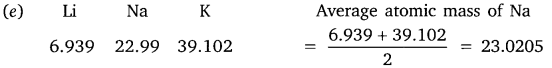The atomic mass of Na is the average atomic mass of Li and K and these elements resemble with each other.

Question 2.
(a) Why do we classify elements?
(b) What were the two criteria used by Mendeleev in creating his Periodic Table?
(c) Why did Mendeleev leave some gaps in his Periodic Table?
(d) In Mendeleev’s Periodic Table, why was there no mention of Noble gases like Helium, Neon and Argon?
(e) Would you place two isotopes of chlorine Cl-35 and Cl-37 in different slots because of their different atomic masses or in the same slot because their chemical properties are the same? Justify your answers.    (AI 2008)
(a) Classification is done to study the properties of elements conveniently.
(b) Increasing order of atomic mass and similarity in chemical properties i.e. the nature of oxide and hydride formed.
(c) The gaps were left for undiscovered elements then.
(d) Noble gases were not invented at that time.
(e) Cl – 35 and Cl – 37 will be kept in the same slot as their chemical properties are same.

Question 3.
(a) What are ‘groups’ and ‘periods’ in the Periodic Table?
(b) Two elements M and N belong to groups I and II respectively and are in the same period of the Periodic Table. How do the following properties of M and N vary?
(i) Sizes of their atoms.
(ii) Their metallic characters.
(iii) Their valencies in forming oxides.
(iv) Molecular formulae of their chlorides.    (Foreign 2009)
(a) The vertical column in the Periodic Table are called ‘groups’, the horizontal rows in the table are called periods.

(b) (i) ‘M’ and ‘N’ belong to the same period but group I and II respectively. N is smaller than M as the atomic size decreases on moving from left to right across the Periodic Table.
(ii) M is more metallic than ‘N’ because metallic character decreases from left to right as tendency to lose electrons decreases due to decrease in atomic size.
(iii) The valencies of M and N are 1 and 2 respectively, valency across the period first increases then decreases.
(iv) MCl and NCl2.

Question 4.
(a) Which 2 criteria did Mendeleev use to classify the elements in his table?
(b) State Mendeleev’s Periodic law.
(c) Why could no fixed position be given to hydrogen in Mendeleev’s Periodic Table?
(d) How and why does the atomic size vary as you go:
(i) From left to right along a period
(ii) Down a group?   (CBSE 2009)
(a) Increasing order of atomic mass and similarities in chemical properties of elements i.e., the formula of oxides and hydrides formed by elements.
(b) Mendeleev’s Periodic Law → Properties of elements are periodic functions of their atomic masses.

(c) Hydrogen had no fixed position in Mendeleev’s Periodic table because it resembles alkali metals by forming positive ions and resembles halogens by forming diatomic molecule.

(d) (i) Atomic size decreases from left to right, as the valence electrons are attracted by the nucleus due to increase in the nuclear force.
(ii) The atomic size increases from top to bottom in a group because -the number of shells keep on increasing therefore distance between nucleus and valence electrons increases.

Question 5.
(a) Why did Mendeleev left gaps in his Periodic Table?   (CBSE 2009)
(b) State any 3 limitations of Mendeleev’s classification.
(c) How does electronic configuration of atoms change in period with increase in atomic number?
(a) Mendeleev left some gaps for undiscovered elements, because he predicted that there would be such elements which will fit in the gaps in future. He also predicted the properties of these elements.
(b) 3 limitations are:

1. Position of hydrogen was not justified.
2. Increasing order of atomic mass could not be maintained.
3. Isotopes were not given separate place as they have different atomic mass.

(c) In a period the valence electrons goes on increasing from left to right as the number of shells is same.

Question 6.
An element ‘X’ (Atomic number = 20) burns in the presence of oxygen to form a basic oxide.   (Foreign 2016)
(a) Identify the element and write its electronic configuration.
(b) State its group number and period number in the Modern Periodic Table.
(c) Write a balanced chemical equation for the reaction when this oxide is dissolved in water.